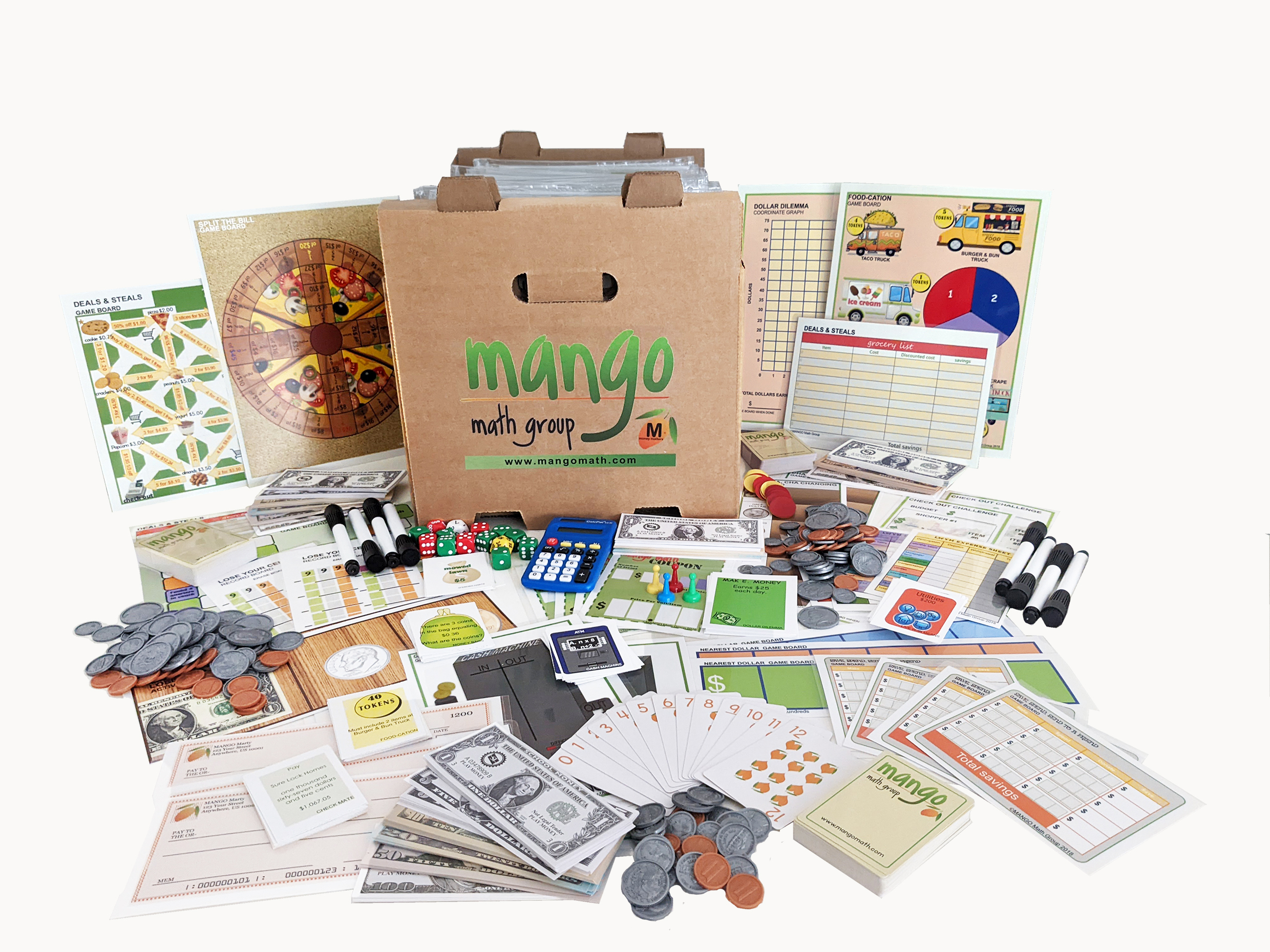#### Order Notice

As the economy opens back up, many of our suppliers are experiencing shortages of parts and raw materials, resulting in delays in shipments. As a result, we anticipate that it may take up to 2-4 weeks from the date we receive a purchase order for us to fulfill and ship any order for our products. We apologize for any inconvenience.

NEW!!

# Money Matters Kit 3rd-5th

Managing money is a necessary life skill, so start students off early with this fun and easy math kit! MANGO Math Money Matters Math kit is designed specifically for third, fourth and fifth grades. The kit contains 20 separate skill building math lessons.

\$ 479.00 USD##### Product Details

Managing money is a necessary life skill so start students off early with this fun and easy math kit! MANGO Math Money Matters Math kit is designed specifically for third, fourth and fifth grades. The kit contains 20 separate skill building math lessons.

Each individual lesson offers visual and kinetic representation of math concepts that develop strong math problem solving strategies, like:

• currency counting
• budgeting, credit
• debit, savings
• check writing
• simple understanding of economics

Every lesson is based on a game or activity that fosters curiosity, discussion and growth through cooperation, collaboration and creative thinking.

MANGO Math's Strong Evidence Based Kits come with individually packaged, Grab-n-Go lessons that includes instructions, reusable materials pre-packaged in a resealable pouch contained in an easy to carry crate that can be used year after year, providing long term savings. A lesson can be used by up to 4 students, set up as math centers with each grade level math kit meeting the needs of 30+ students at a time. Each MANGO Math grade level math kit adheres to Common Core, TEKS and NCTM math standards and is research- based.

Great to use in regular classrooms, resource rooms, after school programs, STEM programs, libraries, math centers, summer camps, learning cooperatives, math clubs, community centers, and at home.

#### All the supplies below are included in this pack:

• Playing paper money
• Play coin money
• Dice
• Pawns
• Bi-colored counters
• Spinner
• MANGO Math playing cards
• 12-side dice
• 10s dice
• Plastic resealable pouches
• Game boards
• Laminated writing boards
• Dry erase markers
• Easy to follow Directions
• Curriculum guide

## Money Matters (3rd – 5th grade) Lessons and Standards

Better Deal – Number Sense & Operations: Students will divide single divisor into a single or multiple-digit dividends.

Cash Machine – Algebraic Thinking: Students will generate numerical patterns using two given rules.  Identify apparent relationship between terms.

Check Mate – Number Sense: Students will write numbers in written and numerical form.  Understand the process of writing checks.

Check Out Challenge – Measurement: Students will use additional and subtraction to solve word problems involving money including simple decimals.

Coin a Phrase – Number Sense: Students will skip count by 100s, 25s, 10s, 5s, and 1s like that used in counting change.

Cha, Cha, Cha Changing – Number Sense: Students will solve problems involving quarters, dimes, nickels, and pennies.

Deals and Steals – Operations & Fractions: Students will divide amounts, multiply simple fractions, and percent to create a single item’s dollar amount.

Dollar Dilemma – Number Sense: Solve multistep word problems posed with whole numbers and having whole-number answers using the four operations.

Food-cation – Number Sense: Multiply or divide to solve word problems involving multiplicative comparison, using symbol for the unknown number.

Living within your Means – Algebraic Thinking: Students will be given occupations and expenses and allocated monthly expense.

Lose Your Cents – Number Sense: Students will understand subtraction of numbers based on hundreds, tens and ones; how to compose or decompose tens or hundreds.

Money Bags – Algebraic Thinking: Students will model algebraic thinking to solve the problem.  Understand value of money.

Nearest Dollar – Number Sense: Students will use place value understanding to round to the nearest dollar amount.

Profit/Loss – Number Sense: Students will collect money and play the odds of saving it or losing it.  Adding and subtracting money.

Push the Envelope – Operations & Base Ten: Students will understand that two or three digits in a number represents amount of hundreds, tens and ones.

Save, Spend, Send – Number Sense: Students will develop place value, addition and subtraction skills in addition some understanding negative numbers.

Spending Habits – Number Sense & Operations: Students will develop an understanding of positive and negative numbers as it pertains to debit and credit.

Split the Bill – Number Sense and Fractions: Students will multiply fractions to figure out their portion of a bill.

Tip Jar – Number Sense and Fractions: Students will divide decimal numbers.

Trade War – Algebraic Thinking: Students will develop an understanding of availability or scarcity of resources and how that impacts cost.

Width
12
in
Height
12
in
Length
18
in
Weight
20
lb